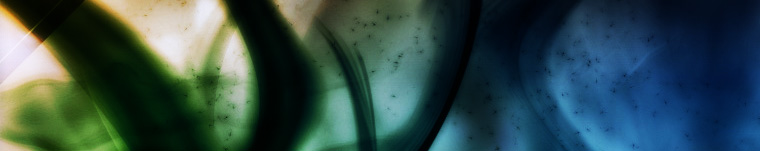## A method to approximate the distribution of Prime numner

The idea is following my idea to find all the factors of a number. We know that for a number X, all its factor could be found within square of X. So for  N^2, at most it will have N factors, or it will have none except 1 and N.

Since we also know that the factor of 2 has a probability of 1/2 in any natural number; the factor of 3 has a probability of 1/3 in any natural number; thereby the factor N has a probability of 1/N in any natural number. We thus can find the prime by eliminate all known factors.

To count the prime number within range of 1 to N^2 is thus,

[1-(1/2+1/3+1/4+…+1/N-(Any combinations of 1/2,1/3….1/N))]*N

Now consider the various range:
For N=2, the probability of a prime=1-1/2=1/2,
which is 2.
For N=3, the probability of a prime=1-(1/2+1/3-1/6)=1/3,
the prime numbers are 2,3,5,7 which is 4/9;
there is a difference of 1/9.

How come? Because when counting prime numbers, we discount 2,3 because they are also the multiple of 2 and 3.

We can assume that as N become greater, the different would be smaller.

~ 由 newnewhkcc1976 於 20 四月, 2009.